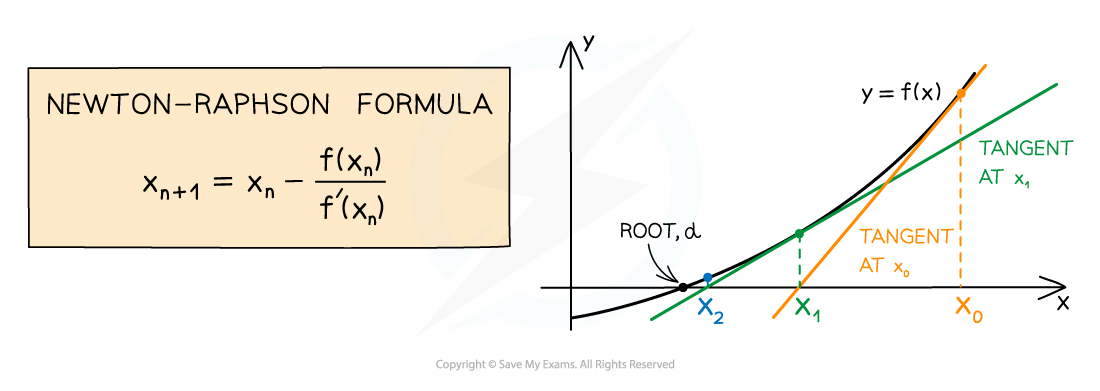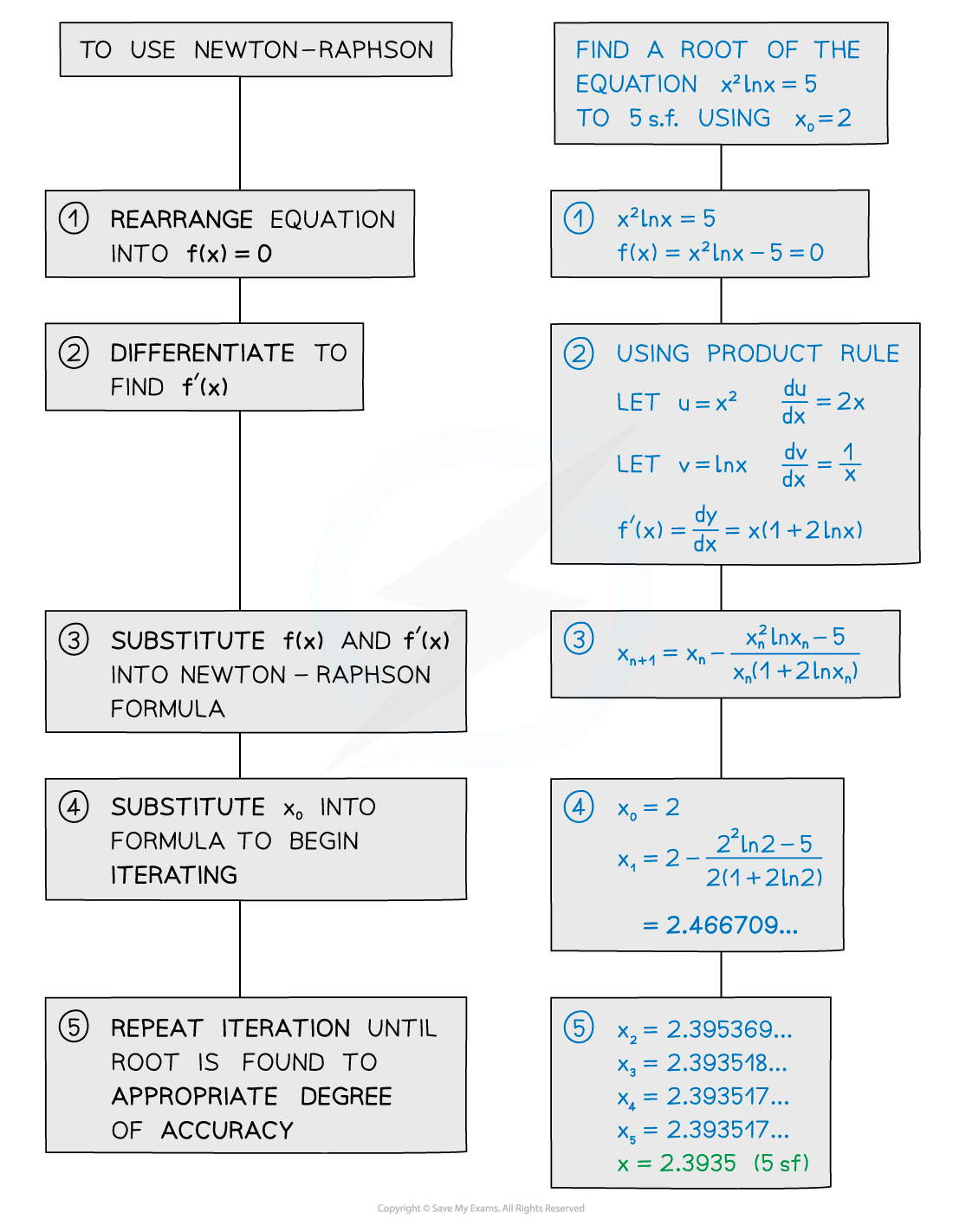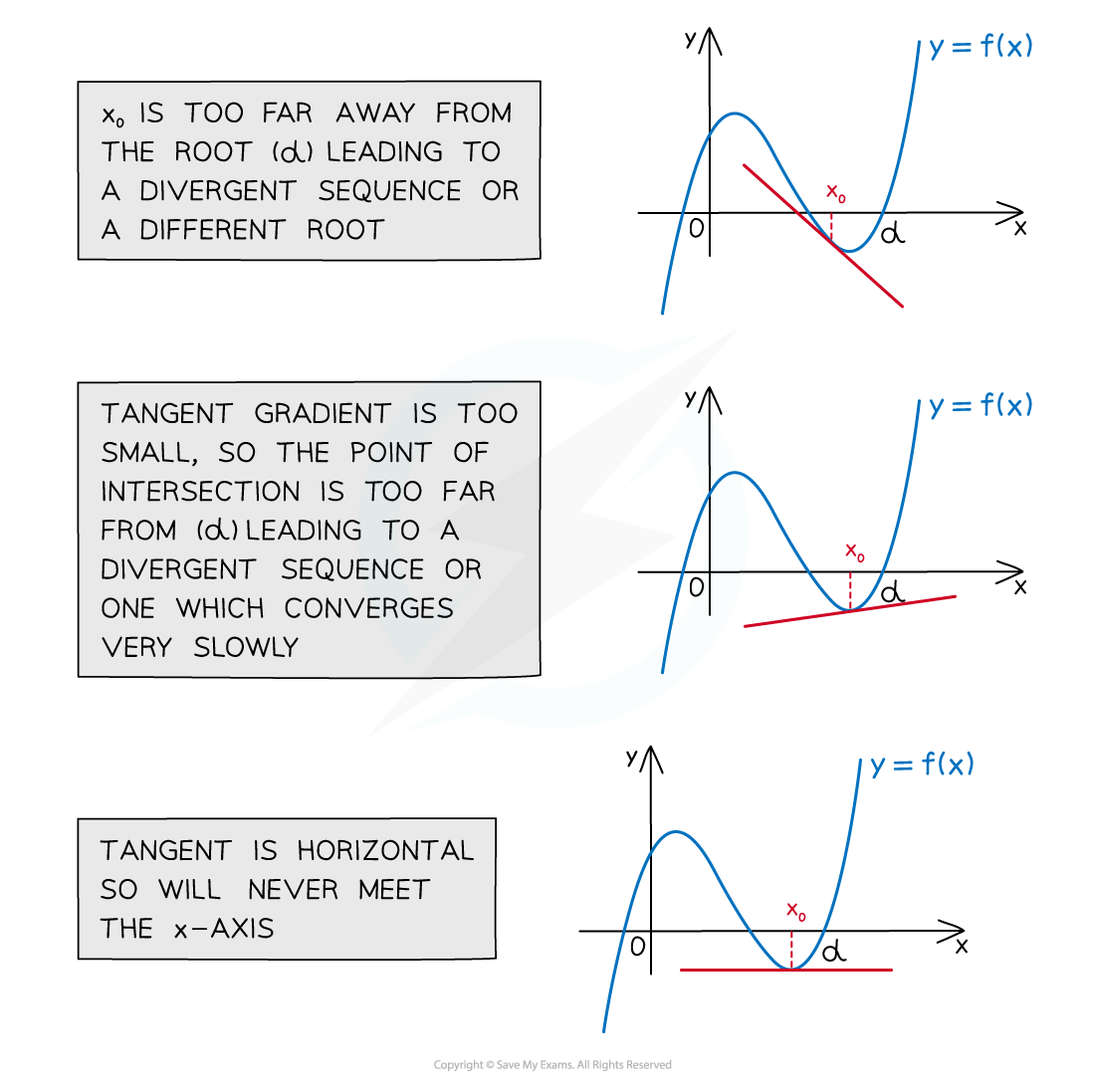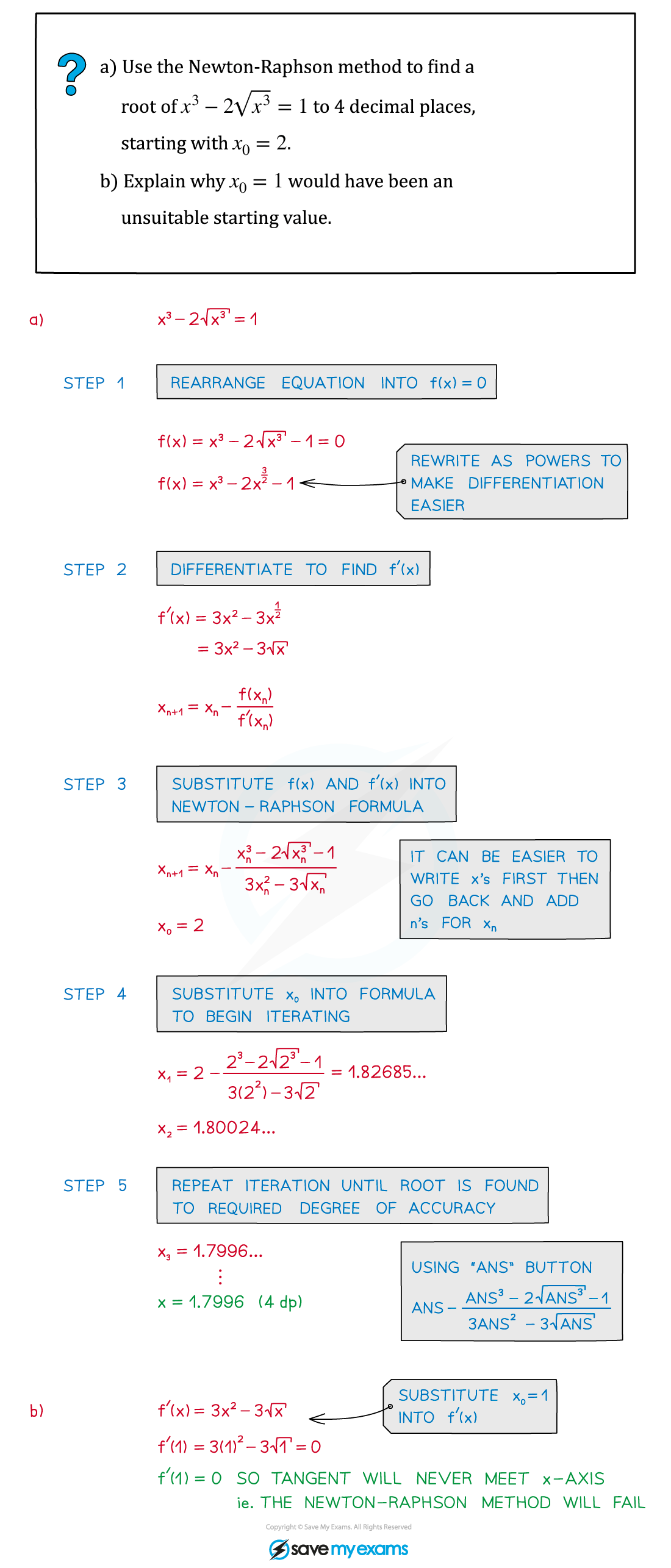# AQA A Level Maths: Pure复习笔记10.1.4 Newton-Raphson

### Newton-Raphson

#### The Newton-Raphson method

• The Newton-Raphson method finds roots of equations in the form f(x) = 0
• It can be used to find approximate solutions when an equation cannot be solved using the usual analytical methods
• It works by finding the x-intercept of tangents to f(x) to get closer and closer to a root#### Using the Newton-Raphson method

• The formula for Newton-Raphson uses the same xn + 1 = f(xn) notation as used in iteration and other recurrence relations
• After using differentiation to find f’(x) the formula uses iteration to come to an ever more accurate solution#### Can the Newton-Raphson method fail?

The Newton-Raphson method can fail when:

• the starting value x0 is too far away from the root leading to a divergent sequence or a different root
• the tangent gradient is too small, where f’(x) close to 0 leading to a divergent sequence or one which converges very slowly
• the tangent is horizontal, where f’(x) = 0 so the tangent will never meet the x‑axis
• the equation cannot be differentiated (or is awkward and time-consuming to do)#### Exam Tip

• The formula for the Newton-Raphson method is given in the formula booklet.
• Use ANS button on your calculator to calculate repeated iterations.
• Keep track of your iterations using x2, x3… notation.
• Newton-Raphson questions may be part of bigger numerical methods questions.

#### Worked Example